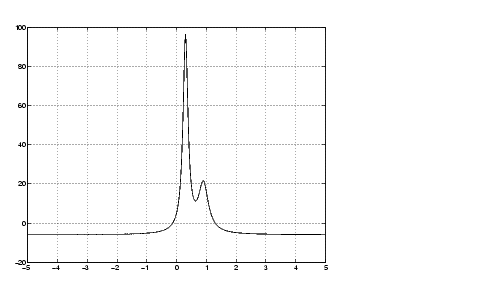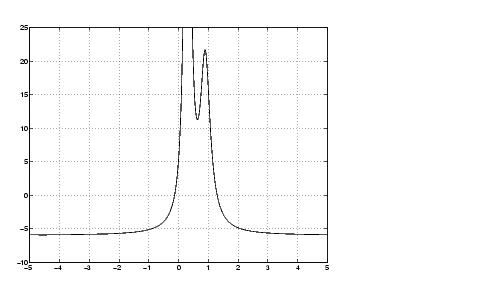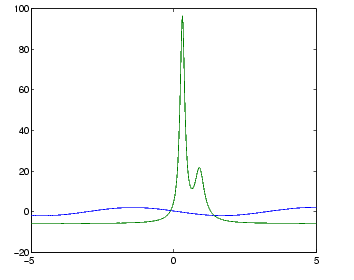MathematicsPlotting Mathematical Functions

The `fplot` function plots a mathematical function between a given set of axes limits. You can control the x-axis limits only, or both the x- and y-axis limits. For example, to plot the `humps` function over the x-axis range `[-5 5]`, use

• ```fplot(@humps,[-5 5])
grid on```

You can zoom in on the function by selecting y-axis limits of `-10` and `25`, using

• ```fplot(@humps,[-5 5 -10 25])
grid on```

You can also pass the function handle for an anonymous function for `fplot` to graph, as in

• ```fplot(@(x)2*sin(x+3),[-1 1]);
```

You can plot more than one function on the same graph with one call to `fplot`. If you use this with a function, then the function must take a column vector `x` and return a matrix where each column corresponds to each function, evaluated at each value of `x`.

If you pass an anonymous function consisting of several functions to `fplot`, the anonymous function also must return a matrix where each column corresponds to each function evaluated at each value of `x`, as in

• ```fplot(@(x)[2*sin(x+3), humps(x)],[-5 5])
```

which plots the first and second functions on the same graph.Note that the anonymous function

• ```fh = @(x)[2*sin(x+3), humps(x)];
```

evaluates to a matrix of two columns, one for each function, when `x` is a column vector.

• ```fh([1;2;3])
```

returns

• ```   -1.5136   16.0000
-1.9178   -4.8552
-0.5588   -5.6383
```Representing Functions in MATLAB Minimizing Functions and Finding Zeros© 1994-2005 The MathWorks, Inc.